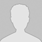>> 更多

### DedeCMS修改水印坐标调整水印位置教程

DedeCMS 系统默认水印的位置与图片边线相隔5像素,因此追求美观时需要修改水印的坐标,实现方法如下: 找到include目录下的image.class.php文件，查找以下代码： 查看源码 打印代码帮助 01 x = +5; 02 03 \$y = +5; 04 05 break ; 06 07 case 2: 08 09 \$x = (\$imagewidth - \$logowidth) / 2; 10 11 \$y = +5; 12 13 break ; 14 15 case 3: 16 17 \$x = […]

DedeCMS系统默认水印的位置与图片边线相隔5像素,因此追求美观时需要修改水印的坐标,实现方法如下:

 `01` `x = +5;`
 `02`
 `03` `\$y = +5;`
 `04`
 `05` `break``;`
 `06`
 `07` `case` `2:`
 `08`
 `09` `\$x = (\$imagewidth - \$logowidth) / 2;`
 `10`
 `11` `\$y = +5;`
 `12`
 `13` `break``;`
 `14`
 `15` `case` `3:`
 `16`
 `17` `\$x = \$imagewidth - \$logowidth - 5;`
 `18`
 `19` `\$y = +5;`
 `20`
 `21` `break``;`
 `22`
 `23` `case` `4:`
 `24`
 `25` `\$x = +5;`
 `26`
 `27` `\$y = (\$imageheight - \$logoheight) / 2;`
 `28`
 `29` `break``;`
 `30`
 `31` `case` `5:`
 `32`
 `33` `\$x = (\$imagewidth - \$logowidth) / 2;`
 `34`
 `35` `\$y = (\$imageheight - \$logoheight) / 2;`
 `36`
 `37` `break``;`
 `38`
 `39` `case` `6:`
 `40`
 `41` `\$x = \$imagewidth - \$logowidth - 5;`
 `42`
 `43` `\$y = (\$imageheight - \$logoheight) / 2;`
 `44`
 `45` `break``;`
 `46`
 `47` `case` `7:`
 `48`
 `49` `\$x = +5;`
 `50`
 `51` `\$y = \$imageheight - \$logoheight - 5;`
 `52`
 `53` `break``;`
 `54`
 `55` `case` `8:`
 `56`
 `57` `\$x = (\$imagewidth - \$logowidth) / 2;`
 `58`
 `59` `\$y = \$imageheight - \$logoheight - 5;`
 `60`
 `61` `break``;`
 `62`
 `63` `case` `9:`
 `64`
 `65` `\$x = \$imagewidth - \$logowidth - 5;`
 `66`
 `67` `\$y = \$imageheight - \$logoheight -5;`
 `68`
 `69` `break``;`• 全部评论（0
还没有评论，快来抢沙发吧！